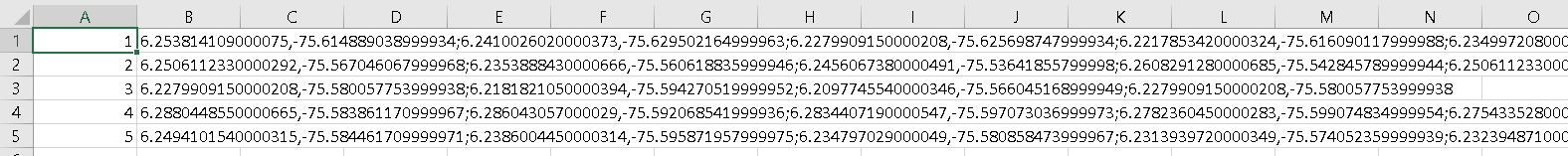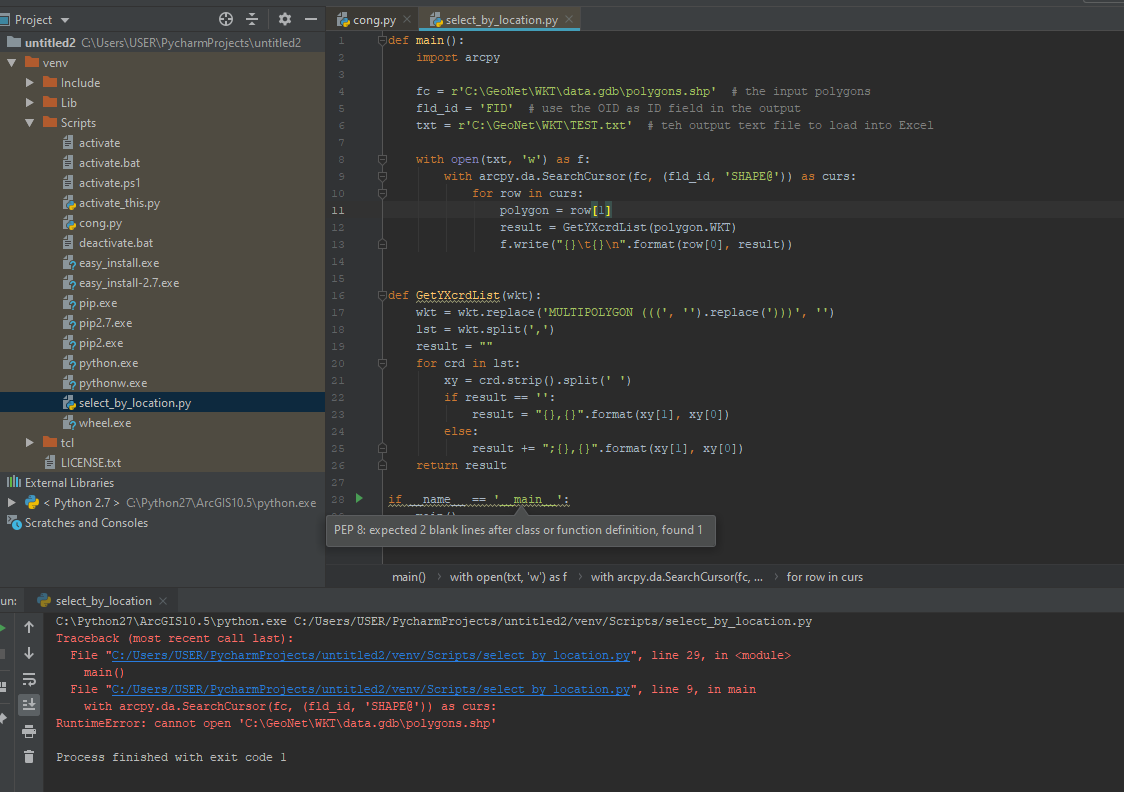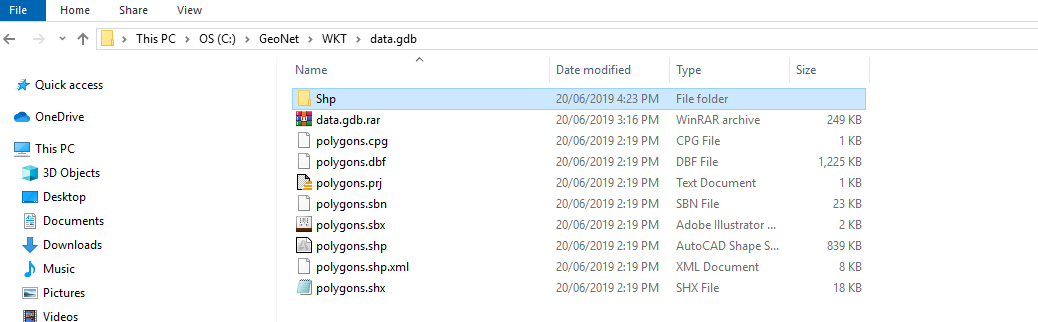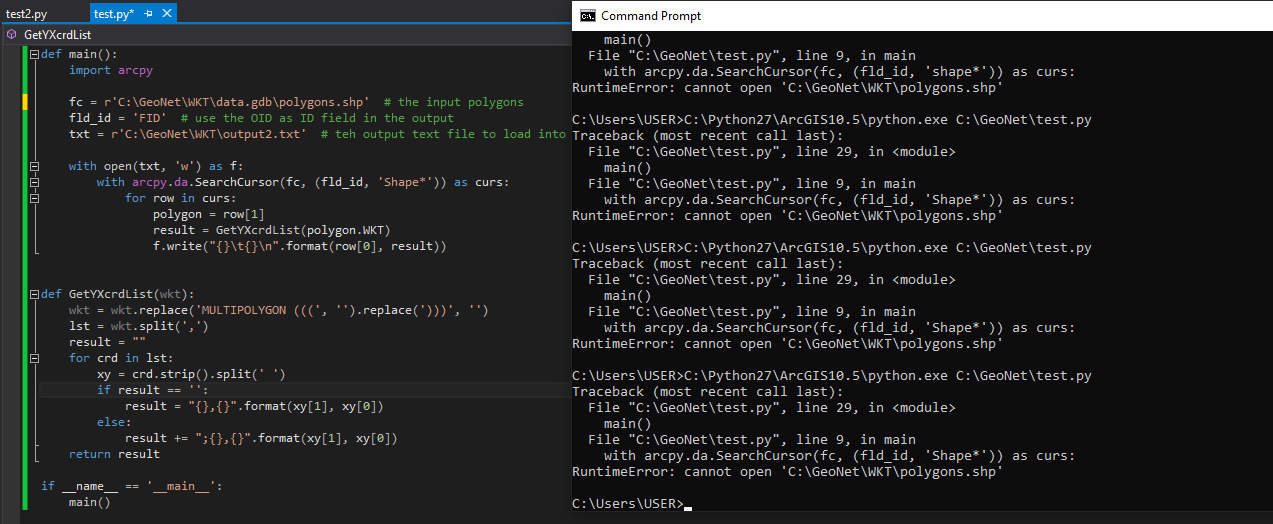# How do I export cordinates of polygon shapes to excel.

9186
14
03-13-2017 06:14 AMNew Contributor

I received shape files, and imported it.  I can see the shapes, but I need to export the cordinate points of the shape to an excel or csv file in order to import into another program.

14 RepliesbyMVP Esteemed Contributor

If you are looking for the XY coordinates of Centroid of polygons, you could add two Fields to Attribute Table, viz, X and Y and use Calculate Geometry to populate the fields with the X and Y coordinate of the Centroid. Then you can use Table to Excel geoprocessing tool to export to Excel.

But, if you want the XY coordinates of all the vertices of the polygon, you will need to convert feature vertices to points, add the XY fields to attribute table and populate using Calculate Geometry. Then Go for Table to Excel.

Think LocationbyMVP Legendary Contributor

For all points for all license levels, there are other workaroundsThe key is the explode_to_points which busts up the geometry into its constituent points.  Rows 1 to 5 form the clockwise points of a square in this example.  Once busted up, bring it back in as table and export to excel, or there are tools to simply send the array to excel directly...New Contributor III

Hi Dan,

Is it possible to export the coordinates of vertices of a polygon into excel or as a text file in the following format: Polygon1: y,x ; y,x ;y,x ; y,x

Therefore, all coordinates that make up a polygon should be in one row?byMVP Legendary Contributor

Liesl Not without coding the output to from numpy to a row format.  If you have a use case, you might want to start another question describing the need to go to excel and why WKT or similar output format is neededbyEsri Esteemed Contributor

I guess you could do something like this with a bit of Python code:

``````def main():
import arcpy

fc = r'C:\GeoNet\WKT\data.gdb\polygons'  # the input polygons
fld_id = 'OID@'  # use the OID as ID field in the output
txt = r'C:\GeoNet\WKT\output2.txt'  # teh output text file to load into Excel

with open(txt, 'w') as f:
with arcpy.da.SearchCursor(fc, (fld_id, 'SHAPE@')) as curs:
for row in curs:
polygon = row
result = GetYXcrdList(polygon.WKT)
f.write("{}\t{}\n".format(row, result))

def GetYXcrdList(wkt):
wkt = wkt.replace('MULTIPOLYGON (((', '').replace(')))', '')
lst = wkt.split(',')
result = ""
for crd in lst:
xy = crd.strip().split(' ')
if result == '':
result = "{},{}".format(xy, xy)
else:
result += ";{},{}".format(xy, xy)
return result

if __name__ == '__main__':
main()
‍‍‍‍‍‍‍‍‍‍‍‍‍‍‍‍‍‍‍‍‍‍‍‍‍‍‍‍‍``````

Just change:

• line 4 to point to your polygon featureclass
• line 5 to include a field to identify the resulting coordinate lists
• line 6 to point to an output text file that can be loaded into Excel

Below the result of a test run:What do you want to do with this list of Lat, Lon coordinates in Excel?by
New Contributor IIIi cant run this code

pls help me with this error @Xander BakkerbyMVP Legendary Contributor

You have a shapefile in a geodatabase... try a featureclass in a geodatabase

You have to edit the full path to the inputs... you just changed "Polygons" to polygons.shp in Xander's code on line 4, so unless you recreated his folder path structure, it won't workby
New Contributor III

i have ald changed folder path structure , but it didn't work. .

it is my link folder , i make like himthe error still just say: "cannot open"